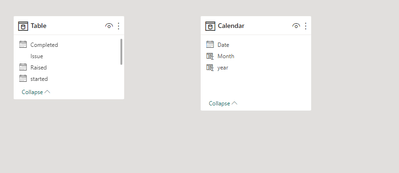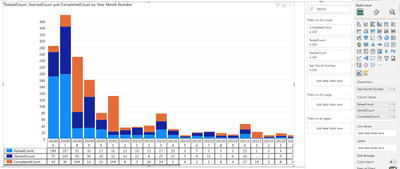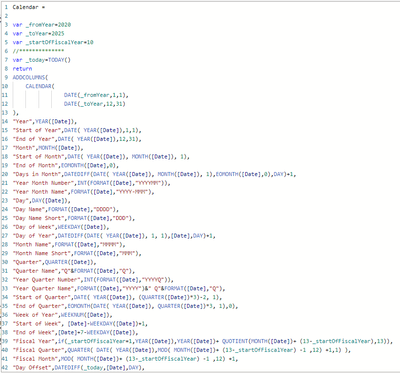cancel
Showing results for
Did you mean:Helper II

## Create A Stacked Column Chart that Shows Monthly Counts

I have a simple table of data that shows an "Issue_#" and three dates for each row. All I want to be able to do is create a stacked column chart that will show the number of "Issue_#"s that were "Raised", "Started" and "Completed" each Year and Month with the respective breakdowns.

I have tried building a calendar table and making the relatioships, but nothing I seem to do gives me the right information. Can someone provide a simple step by step explanation to what I need to do to make this work?2 ACCEPTED SOLUTIONSCommunity Champion

Hi @mkusler
You can achieve it with disonacted date table and 3 dax dormulas for evrery "status":1.

StartedCount =
var start_ = min('Calendar'[Date])
var end_ =max('Calendar'[Date])
var starteD_count = CALCULATE(DISTINCTCOUNT('Table'[Issue]),
'Table'[started]>=start_ && 'Table'[started]<=end_)
return starteD_count
2.
RaisedCount =
var start_ = min('Calendar'[Date])
var end_ =max('Calendar'[Date])
var Rased_count = CALCULATE(DISTINCTCOUNT('Table'[Issue]),
'Table'[Raised]>=start_ && 'Table'[Raised]<=end_)
return Rased_count
3.
CompletedCount =
var start_ = min('Calendar'[Date])
var end_ =max('Calendar'[Date])
var _count = CALCULATE(DISTINCTCOUNT('Table'[Completed]),
'Table'[Completed]>=start_ && 'Table'[Completed]<=end_)
return _count
Result :If this post helps, then please consider Accepting it as the solution to help the other members find it more quicklyCommunity Champion

Hello, I didn't quite understand the format of the date you displayed.
In general, you should create a numeric column that allows you to sort the column you use on your graph from the calendar table.
Here is an example from my answer in another discussion, you should use the same logic, refer to your format:
https://community.fabric.microsoft.com/t5/Desktop/Trend-lines-Month-Name-by-year-are-not-in-ascendin...

If this post helps, then please consider Accepting it as the solution to help the other members find it more quickly

5 REPLIES 5Helper II

@Ritaf1983 Thank you again! That was a simple solution. I didn't even think of adding in a sort column. All is good now!Community Champion

I was happy to assist 🙂Community Champion

Hi @mkusler
You can achieve it with disonacted date table and 3 dax dormulas for evrery "status":1.

StartedCount =
var start_ = min('Calendar'[Date])
var end_ =max('Calendar'[Date])
var starteD_count = CALCULATE(DISTINCTCOUNT('Table'[Issue]),
'Table'[started]>=start_ && 'Table'[started]<=end_)
return starteD_count
2.
RaisedCount =
var start_ = min('Calendar'[Date])
var end_ =max('Calendar'[Date])
var Rased_count = CALCULATE(DISTINCTCOUNT('Table'[Issue]),
'Table'[Raised]>=start_ && 'Table'[Raised]<=end_)
return Rased_count
3.
CompletedCount =
var start_ = min('Calendar'[Date])
var end_ =max('Calendar'[Date])
var _count = CALCULATE(DISTINCTCOUNT('Table'[Completed]),
'Table'[Completed]>=start_ && 'Table'[Completed]<=end_)
return _count
Result :If this post helps, then please consider Accepting it as the solution to help the other members find it more quicklyHelper II

That worked! Thank you!

One more question.....I can't seem to get my visual to sort the year/month correctly, and also there is another "0" being added after each year. Do you know how to fix this? I have tried changing the sort criteria, but it's always jumbled.Is there something wrong with my calendar table setup for "Year Month Number"?Thanks again!Community Champion

Hello, I didn't quite understand the format of the date you displayed.
In general, you should create a numeric column that allows you to sort the column you use on your graph from the calendar table.
Here is an example from my answer in another discussion, you should use the same logic, refer to your format:
https://community.fabric.microsoft.com/t5/Desktop/Trend-lines-Month-Name-by-year-are-not-in-ascendin...

If this post helps, then please consider Accepting it as the solution to help the other members find it more quickly